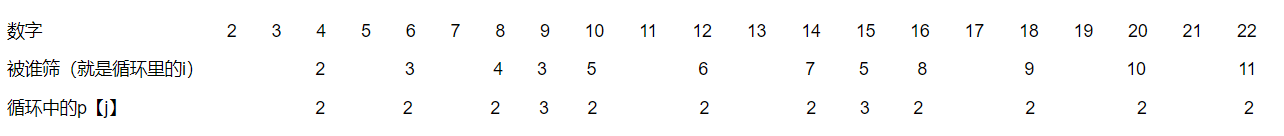# 线性筛法（欧拉筛法）求素数 和 质因数分解

for(i = 2; i <= n; i++)
{
if(f[i] == 0)
{
p[++cnt] = i;
}
for(j = 1; j <= cnt; j++)
{
if(i * p[j] > n)break;因为求n以内质数。
f[i * p[j]] = 1;
if(i % p[j] == 0)break;//这句话是关键。这句话保证了一个数被他最大的因数（除自己本身）筛。。。也可以说是被最小的质因数筛。
}
}

手推一下可以清晰理解。。。我来写一下。。。。就比如12是被2  6  筛掉的，而3    4  并没有去筛12       就是因为

if(i % p[j] == 0)break;这句话保证了一个数被他最大的因数（除自己本身）筛。

4筛完8   因为4%2==0；   打断当前循环，没有去筛12.

需要加的就是记录一下被哪个质数筛的。

 if(f[i] == 0)
{
p[++cnt] = i;        s[i]=i;
}
f[i * p[j]] = 1;s[i*p[j]]=p[j];这两处写为这样，s记录的就是一个数的最小质因数，那么分解质因数时只需要不断去除当前数的最小质因数，知道除到1，质因数便分解完了。

posted @ 2016-09-25 16:52  xuzc  阅读(3437)  评论(0编辑  收藏  举报